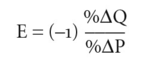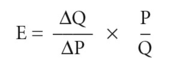# point elasticity

(redirected from Elasticity of a function)
Also found in: Wikipedia.

## point elasticity

a precise measure of the responsiveness of DEMAND or SUPPLY to changes in PRICE, INCOME, etc. Taking PRICE-ELASTICITY OF DEMAND, point elasticity may be defined as:where E = price-elasticity of demand, %δQ = percentage change in quantity demanded, P = percentage change in price.

Since the DEMAND CURVE slopes downwards from left to right, the δ Q has the opposite sign to ZiP, so (-1) is added to the equation to generate a positive value for the elasticity figure. The numerical value of E signifies the degree of elasticity, that is, it lies between zero and infinity. An example of the measurement of point elasticity is given in Fig. 143.

In order to measure elasticity at a particular price, P, we need to measure the slope of the demand curve at point T where the demand curve is tangential to the straight line LL. The slope of the tangent LL is equal to δQ/A P where the increment in output (^ Q) is very small. This gives the formula for point elasticity:Where the calculated value of E is greater than 1, demand is described as elastic; where E is less than 1, but greater than zero, demand is inelastic. See also ARC ELASTICITY.

Collins Dictionary of Economics, 4th ed. © C. Pass, B. Lowes, L. Davies 2005
Site: Follow: Share:
Open / Close